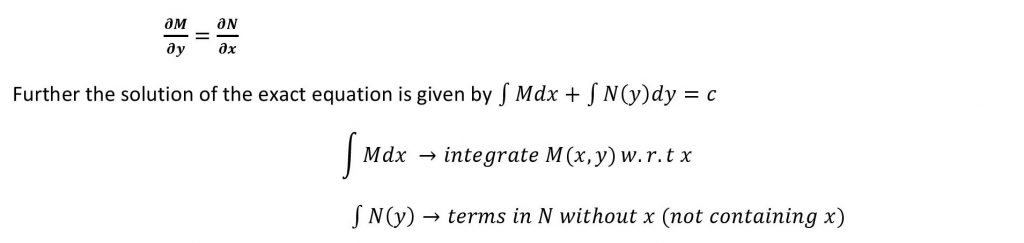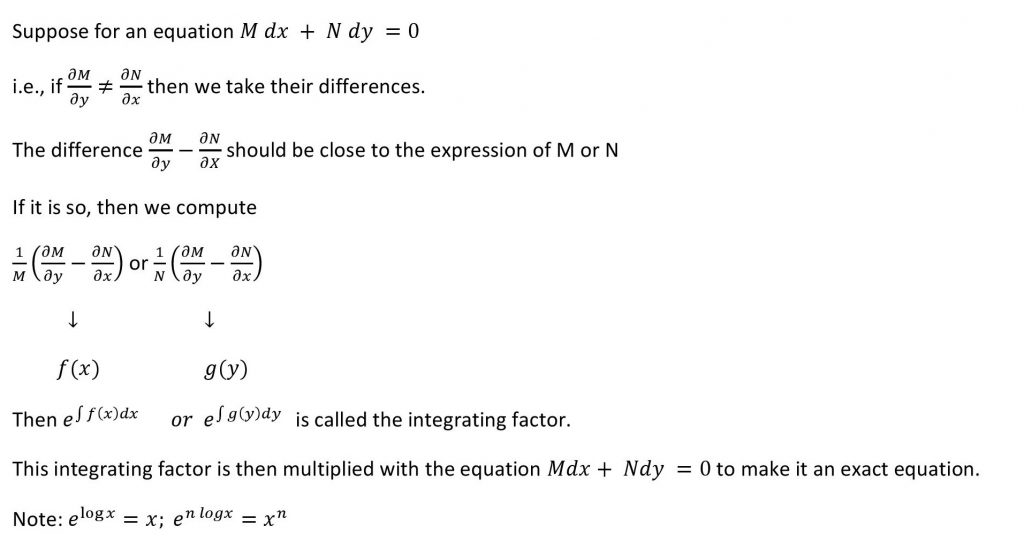## Exact Differential Equations

An exact differential equation is a type of ordinary differential equation (ODE) in which the total differential of a function is equal to the product of a function and a differential. Exact differential equations have the property that their solutions are unique, provided suitable initial or boundary conditions are specified.

## TYPE 1

For any given function f(x,y), the necessary and the sufficient condition for the differential equations M (x,y)dx+N(x,y)dy=0 to be an exact equation is## Type 2

EQUATIONS REDUCIBLE TO THE EXACT FORM

An ordinary differential equation (ODE) is said to be reducible to the exact form if it can be written as an exact differential equation. In other words, the ordinary differential equation (ODE) which is not an exact equation can be transformed into an exact equation by multiplying with some function (factor) known as integrating factor (IF)